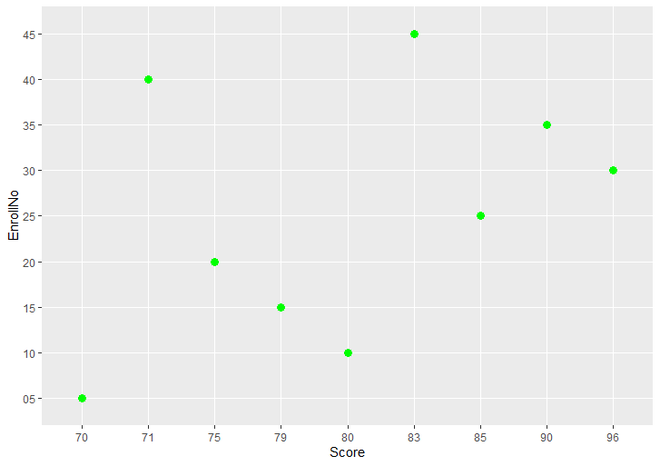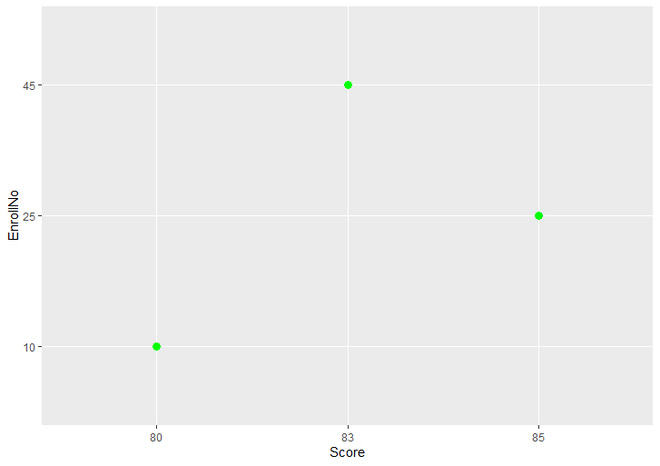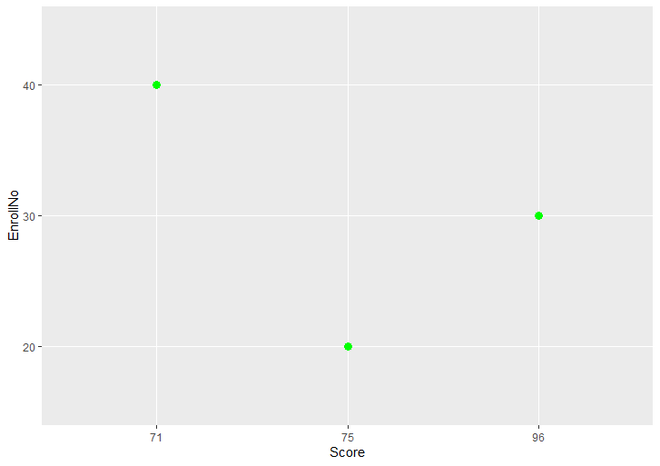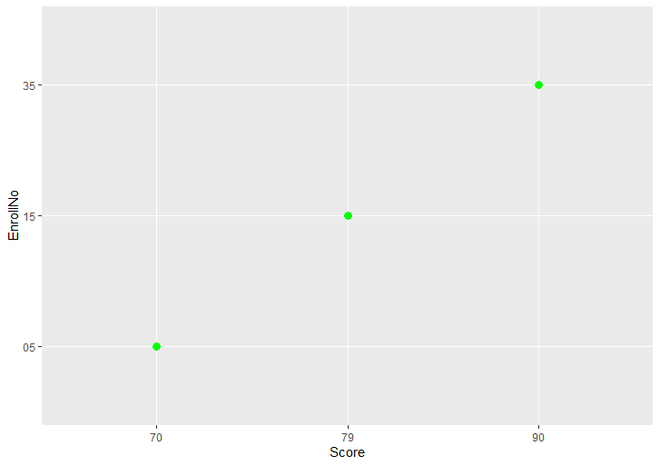# How to plot a subset of a dataframe using ggplot2 in R ?

• Last Updated : 16 May, 2021

In this article, we will discuss how to plot a subset of a dataframe using ggplot2 in the R programming language.

Dataframe in use:

To get a complete picture, let us first draw a complete dataframe.

Example:

## R

 `# Load ggplot2 package``library``(ggplot2)`` ` `# Create Data For plotting``Age <- ``c``(``"17"``, ``"18"``, ``"17"``, ``"19"``, ``"18"``, ``"19"``,``         ``"17"``, ``"19"``, ``"18"``)`` ` `EnrollNo <- ``c``(``"05"``, ``"10"``, ``"15"``, ``"20"``, ``"25"``,``              ``"30"``, ``"35"``, ``"40"``, ``"45"``)`` ` `Score <- ``c``(``"70"``, ``"80"``, ``"79"``, ``"75"``, ``"85"``, ``"96"``,``           ``"90"``, ``"71"``, ``"83"``)`` ` `# Create a DataFrame from Data``data <- ``data.frame``(Age, EnrollNo, Score)`` ` `# Create a simple Scatter Plot``ggplot``(data, ``aes``(Score, EnrollNo)) + ``  ``geom_point``(color = ``"green"``, size = 3)`

Output:Simple Scatter Plot

Now, we will see all methods to create an R Plot using subset of DataFrame one by one.

### Method 1: Using subset() function

Here, we use subset() function for plotting only subset of DataFrame inside ggplot() function inplace of data DataFrame. All other things are same.

Syntax: subset(obj, …)

Parameters:

• It takes data object to be subsetted as it’s first parameter.
• subset() function can have many other parameters. only obj is necessary to take. here we only use logical expression indicating rows as a second argument.

Return : subset() function returns subset of DataFrame.

Example:

## R

 `# Load ggplot2 package``library``(ggplot2)`` ` `# Create Data For plotting``Age <- ``c``(``"17"``, ``"18"``, ``"17"``, ``"19"``, ``"18"``, ``"19"``,``         ``"17"``, ``"19"``, ``"18"``)`` ` `EnrollNo <- ``c``(``"05"``, ``"10"``, ``"15"``, ``"20"``, ``"25"``, ``              ``"30"``, ``"35"``, ``"40"``, ``"45"``)`` ` `Score <- ``c``(``"70"``, ``"80"``, ``"79"``, ``"75"``, ``"85"``, ``"96"``,``           ``"90"``, ``"71"``, ``"83"``)`` ` ` ` `# Create a DataFrame from Data``data <- ``data.frame``(Age, EnrollNo, Score)`` ` `# Generate R Scatter Plot only where Age ``# variable has value "18"``ggplot``(``subset``(data, Age %``in``% ``"18"``), ``aes``(Score, EnrollNo)) + ``  ``geom_point``(color = ``"green"``, size = 3)`

Output:Scatter Plot using only subset of DataFrame by sunset() function

### Method 2: Using Square Brackets

In this method we are not using subset() function, but we write logical expression for retrieve subset of DataFrame in Square Brackets and assign it to subset variable.

Example:

## R

 `# Load ggplot2 package``library``(ggplot2)`` ` `# Create Data For plotting``Age <- ``c``(``"17"``, ``"18"``, ``"17"``, ``"19"``, ``"18"``, ``"19"``, ``         ``"17"``, ``"19"``, ``"18"``)`` ` `EnrollNo <- ``c``(``"05"``, ``"10"``, ``"15"``, ``"20"``, ``"25"``,``              ``"30"``, ``"35"``, ``"40"``, ``"45"``)`` ` `Score <- ``c``(``"70"``, ``"80"``, ``"79"``, ``"75"``, ``"85"``, ``"96"``,``           ``"90"``, ``"71"``, ``"83"``)`` ` `# Create a DataFrame from Data``data <- ``data.frame``(Age, EnrollNo, Score)`` ` `# Create a Variable 'data_subset' ``# which has the values equal to ``# "19" values of Age object.``data_subset <- data[data\$Age %``in``% ``"19"``, ]`` ` `# Generate R Scatter Plot only where``# Age variable has value "19"``ggplot``(data_subset, ``aes``(Score, EnrollNo)) + ``  ``geom_point``(color = ``"green"``, size = 3)`

Output:Scatter Plot using only subset of DataFrame by Square Brackets

### Method 3: Using Square Brackets inside ggplot() Function

In this method, We use square brackets to write expression for subset of DataFrame, but we use it inside ggplot() function in place of dataframe object.

Example

## R

 `# Load ggplot2 package``library``(ggplot2)`` ` `# Create Data For plotting``Age <- ``c``(``"17"``, ``"18"``, ``"17"``, ``"19"``, ``"18"``, ``"19"``,``         ``"17"``, ``"19"``, ``"18"``)`` ` `EnrollNo <- ``c``(``"05"``, ``"10"``, ``"15"``, ``"20"``, ``"25"``, ``              ``"30"``, ``"35"``, ``"40"``, ``"45"``)`` ` `Score <- ``c``(``"70"``, ``"80"``, ``"79"``, ``"75"``, ``"85"``, ``"96"``, ``           ``"90"``, ``"71"``, ``"83"``)`` ` `# Create a DataFrame from Data``data <- ``data.frame``(Age, EnrollNo, Score)`` ` `# Generate R Scatter Plot only where``# Age variable has value "17"``ggplot``(data[data\$Age %``in``% ``"17"``, ], ``aes``(Score, EnrollNo)) + ``  ``geom_point``(color = ``"green"``, size = 3)`

Output:My Personal Notes arrow_drop_up